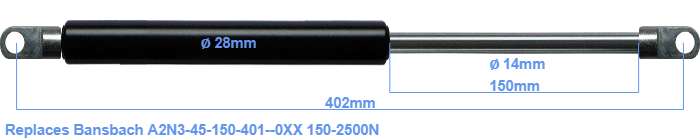# Replacement for Bansbach A2N3-45-150-401–0XX 150-2500N

\$39.74

Replacement gas spring for the Bansbach A2N3-45-150-401--0XX 150-2500 Newton. The eye has a thickness of 10mm and a hole diameter of 8.1mm. Brand: HAHN, a Stabilus company.

Please note:
• Mounting part tube: The thickness of the eye of this replacement is 10mm instead of 8mm.
• Mounting part rod : The thickness of the eye of this replacement is 10mm instead of 18mm.
•  Force Choose an option150 Newton200 Newton250 Newton300 Newton350 Newton400 Newton450 Newton500 Newton550 Newton600 Newton650 Newton700 Newton750 Newton800 Newton850 Newton900 Newton950 Newton1000 Newton1050 Newton1100 Newton1150 Newton1200 Newton1250 Newton1300 Newton1350 Newton1400 Newton1450 Newton1500 Newton1550 Newton1600 Newton1650 Newton1700 Newton1750 Newton1800 Newton1850 Newton1900 Newton1950 Newton2000 Newton2050 Newton2100 Newton2150 Newton2200 Newton2250 Newton2300 Newton2350 Newton2400 Newton2450 Newton2500 NewtonClearThe bore of the house of this replacement gas spring is 28 mm. The diameter of the rod totals 14 mm. The stroke is 150 millimeter long. The total length of this replacement is exactly 402 mm. This is the total distance between the centers of the two mounting parts. Without the mounting parts this gas spring is 348 mm long (thread to thread). This replacement gas spring has a force of 150-2500 Newton. This gas spring can replace a Bansbach gas spring, but is not an orignal Bansbach one. This a gas spring with the brand HAHN. The force (150-2500 N) and dimensions are almost identical to the original.